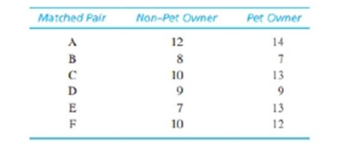Chapter 11, Problem 20PEssentials of Statistics for the B...

8th Edition
Frederick J Gravetter + 1 other
ISBN: 9781133956570

Solutions

Chapter
SectionEssentials of Statistics for the B...

8th Edition
Frederick J Gravetter + 1 other
ISBN: 9781133956570
Textbook Problem

A researcher uses a matched-subjects design to investigate whether single people with pets are happier than singles without pets. A mood inventory survey is given to a group of 20- to 29-year-old non-pet owners and a similar age group of pet owners. The pet owners are matched one to one with the non-pet owners for income, number of close friendships, and general health. The data follow:a. Is there a significant difference in the mood scores for non-pet owners versus pet owners? Test with α = .05 for two tails.  b Construct the 95% confidence interval to estimate the size of the mean difference in mood between the population of pet owners and the population of non-per owners, (You should find that a mean difference of μ D = 0 is an acceptable value, which is consistent with the conclusion from the hypothesis test.)

a.

To determine
Whether there is a significant difference between the mood scores for non-pet owners and pet owners.

Explanation

Given info:

The given data are shown below,

 Matched pair Non pet owner Pet owner A 12 14 B 8 7 C 10 13 D 9 9 E 7 13 F 10 12

Calculation:

The difference D, D and D2 is obtained below:

 Participants White background Red background D D2 A 12 14 2 4 B 8 7 –1 1 C 10 13 3 9 D 9 9 0 0 E 7 13 6 36 F 10 12 2 4 Total 12 54

Thus, the values of D is 14 and D2 is 54.

The mean difference MD:

MD=DN=126=2

Thus, the mean difference MD is 2.

The value of SS is,

SS=D2(D)2N=541446=5424=30

Thus, the value of SS is 30

b.

To determine
The confidence interval to estimate the mood difference at 95 %.

Still sussing out bartleby?

Check out a sample textbook solution.

See a sample solution

The Solution to Your Study Problems

Bartleby provides explanations to thousands of textbook problems written by our experts, many with advanced degrees!

Get Started

What is the defining characteristic of a repeated-measures or within-subjects research design?

Essentials of Statistics for The Behavioral Sciences (MindTap Course List)

CHECKPOINT 1. What is the simple interest formula?

Mathematical Applications for the Management, Life, and Social Sciences

Use the guidelines of this section to sketch the curve. y = (1 x)ex

Single Variable Calculus: Early Transcendentals, Volume I

True or False: converges conditionally.

Study Guide for Stewart's Multivariable Calculus, 8th